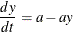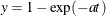# The MODEL Procedure

### Example 19.11 Monte Carlo Simulation

This example illustrates how the form of the error in a ODE model affects the results from a static and dynamic estimation. The differential equation studied isThe analytical solution to this differential equation isThe first data set contains errors that are strictly additive and independent. The data for this estimation are generated by the following DATA step:

data drive1;
a = 0.5;
do iter=1 to 100;
do time = 0 to 50;
y = 1 - exp(-a*time) + 0.1 *rannor(123);
output;
end;
end;
run;


The second data set contains errors that are cumulative in form.

data drive2;
a = 0.5;
yp = 1.0 + 0.01 *rannor(123);
do iter=1 to 100;
do time = 0 to 50;
y = 1 - exp(-a)*(1 - yp);
yp = y + 0.01 *rannor(123);
output;
end;
end;
run;


The following statements perform the 100 static estimations for each data set:

title1 'Monte Carlo Simulation of ODE';

proc model data=drive1 noprint;
parm a 0.5;
dert.y = a - a * y;
fit y / outest=est;
by iter;
run;


Similar statements are used to produce 100 dynamic estimations with a fixed and an unknown initial value. The first value in the data set is used to simulate an error in the initial value. The following PROC UNIVARIATE statements process the estimations:

proc univariate data=est noprint;
var a;
output out=monte mean=mean p5=p5 p95=p95;
run;

proc print data=monte;
run;


The results of these estimations are summarized in Table 19.5.

Table 19.5: Monte Carlo Summary, A=0.5

 Estimation Additive Error Cumulative Error Type mean p95 p5 mean p95 p5 static 0.77885 1.03524 0.54733 0.57863 1.16112 0.31334 dynamic fixed 0.48785 0.63273 0.37644 3.8546E24 8.88E10 -51.9249 dynamic unknown 0.48518 0.62452 0.36754 641704.51 1940.42 -25.6054

For this example model, it is evident that the static estimation is the least sensitive to misspecification.